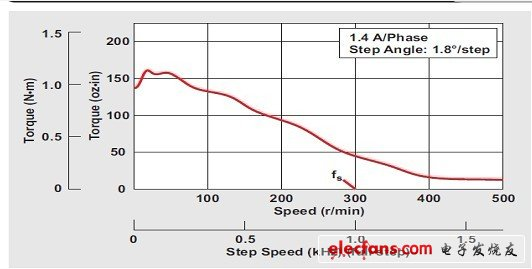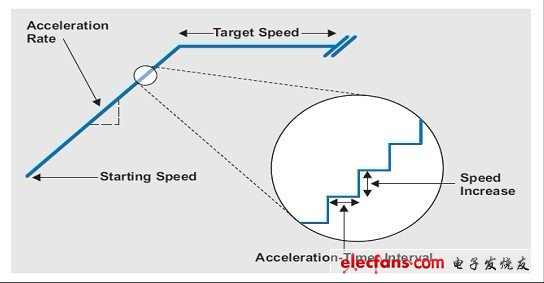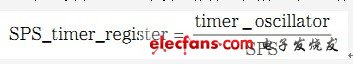# Application of bipolar stepper motor acceleration and deceleration process

As far as DC motors are concerned, by increasing the voltage (or increasing the duty cycle if using pulse width modulation), you can control the speed of the motor drive shaft to a certain speed. However, if it is a stepper motor, changing the voltage will not have any effect on the motor speed. Yes, changing the magnitude of the voltage can change the rate of winding current charge, thereby changing the maximum speed of the stepper motor, but the motor speed is determined by the rate of winding current switching or rectification.

Can we make the assumption that stepper motors are machines that do not require a controlled acceleration process? If it can, then can we let the stepper motor work at any target speed without any care? The fact is that compared to other motor topologies, stepper motors need to be excited through acceleration and deceleration processes. Starting the stepper motor at any speed may have dire consequences.

In this article, we assume that the reader is already familiar with how to use the integrated micro-stepping driver on the market to control the stepping motor. The output of a stepper driver (for example: TI DRV8818, etc.) is proportional to the frequency of the square wave ("step input"). Each "step" pulse is equal to the step defined by the driver's step logic (ie micro-step). Therefore, changing the square wave frequency will also change the speed of the stepper motor accordingly.

Figure 1 shows a traditional step rate / torque curve of a motor manufacturer, where the starting frequency fs is an important parameter. We must know that to start this particular motor normally, a step rate less than fs must be used. Starting the motor with a step rate greater than fs may stop the motor and lose synchronization. Once this happens, the motor rotation control will be seriously affected. On the surface, this is a serious problem, but it is actually easy to solve. All you need to do is start the motor at a step rate below fs and increase the speed until you reach the target speed. Following this principle, the stepper motor can be driven at a step rate that far exceeds fsâ€”as long as the speed remains below the torque / speed curve shown.

Figure 1 Torque / speed curve of bipolar constant current stepping motorIt is also important that the motor cannot be stopped simply by stopping the "step" pulse. On the contrary, the step rate should be reduced from the target speed to a lower rate that can stop the motor without the inertia of the drive shaft, because the inertia of the drive shaft will cause unnecessary and useless stepping. Remember that if a stepper motor is used in positioning applications, the motor drive shaft will lose positioning if the motor continues to rotate when it should stop. Since closed-loop position feedback is rarely used to drive stepper motors, it is important to ensure that only instructional stepping is performed.

Acceleration / Deceleration Process In order to accelerate the stepper motor from the initial speed to a desired target speed, it is only necessary to change the current speed at periodic intervals. Most engineers use microcontrollers to implement stepper motor control. The most common implementation method is to use only two timers. The first is the step per second (SPS) timer, which is used to produce an accurate step rate timing function. The other is the acceleration timer, which is used to periodically change the first timer. Since the speed is periodically changed, the time-dependent angular velocity (dv / dt) is essentially obtained. This process is called acceleration, that is, the speed changes with time. Figure 2 shows an enlarged view of a typical microcontroller-based acceleration profile and describes the process of stepping motor acceleration to a target speed.

Figure 2 Enlarged view of typical acceleration processSPS is the number of steps per second we want to obtain, that is, the step rate of the motor rotation. The SPS timer must be programmed to pulse at this rate. According to the oscillator frequency of the timer, the typical equation is:Among them, SPS_TImer_register is a 16-bit number, which tells the timer how long it takes to generate subsequent "step" pulses, and TImer_oscillator is a constant, which indicates the running speed of the timer in megahertz units.

Store the equation as a function, because it is often used. In order to understand its working process, we assume that the timer oscillator works at 8 MHz and the expected motor step rate is 200 SPS. According to this equation, the program code makes the SPS_TImer_register value equal to 40000. Then, every time the timer counts to 40,000, it generates a "step" pulse. This produces a timer-type output of 200 pulses per second and a 200 SPS shaft rotation.

Each time such an event occurs, an interrupt is generated and the timer is cleared. Timing of the "step" input rising edge is critical to the accuracy of the microstepping driver, but as long as it is before the next "stepping" rising edge, the falling edge will appear almost at any time.

Two parameters are required to define the acceleration curve: (1) SPS value change frequency; (2) SPS value change degree. The acceleration curve is directly proportional to these two parameters; that is, the more frequently the SPS value changes, the larger the value, and the acceleration curve will increase and decrease. The acceleration timer controls these two parameters at the same time: the timer function acts as many times as the SPS value changes per second. In addition, the timer's interrupt service routine (ISR) periodically increases the current SPS by a predetermined factor, thereby Determine the new speed.

Use the step per second per second (SPSPS), or the number of times per second that the current SPS rate changes, to determine the acceleration rate. If the SPS value is changed by increasing 1, the acceleration timer ISR must be called (triggered) every time the acceleration rate changes. For example, when the acceleration rate is 1000 SPSPS, the motor speed starts at 200 SPS and increases periodically by 1 until it reaches 1200 SPS. Then, the ISR of the acceleration timer needs to be called 1000 times.

Another method is to halve the frequency of acceleration timer calls, and then the SPS periodically increases by 2. Compared to the previous example, the ISR of the acceleration timer was only called 500 times, but the motor was still started at 200 SPS and reached 1200 SPS in 1 second. The difference between the two is more real-time availability, but at the expense of reduced resolution. In other words, in order to achieve an accurate acceleration rate of 999 SPSPS, the first method must be used.

There must be a trade-off between the two methods, because your choice determines what kind of motor working quality can be achieved. For example, if you require a lot of granularity to achieve all possible acceleration processes, you need to call the ISR of the acceleration timer as much as possible.

However, in the previous SPS timer equation, there is a division operation. Depending on the processor core used, this division may greatly limit the number of times the ISR is effectively called and a new SPS rate is correctly generated. In an implementation using TI MSP430 â„¢ and a CPU running at 16 MHz, a division operation takes approximately 500 Âµs. As a result, the maximum number of ISR calls per second is 2000. This limitation determines the size of the increment factor. When the acceleration rate is greater than 2000, an increment greater than 1 must be used.

Shortly before the motor starts, an acceleration rate calculation is performed. The software responsible for the calculation determines the time interval and increment factor of the acceleration timer, and then configures the variables accordingly. Use these variables at the same time until the SPS rate is modified enough to reach the target speed. Once the target speed is reached, the acceleration is terminated.

The deceleration process is basically the same as the acceleration process, except that the increment factor is negative instead of positive. In addition, a new target speed at which the motor can safely stop must be specified.

Figure 3 shows an acceleration / deceleration process in which the acceleration and deceleration rates are symmetrical. Asymmetric rates can also be used.

DITUO New Released USB Essential Oil Car Diffuser is 100% PP material. It is the easiest way to maintain a fresh, moisture and odor free environment in your car. USB powered, plug in your car, Car Diffuser is portable to be taken anywhere and fits perfectly to any vehicle cup holder. Two working modes, continuous mist mode and intermittent mist mode. Beautiful 7 colors mood light to relieve tiredness while driving. You have the option of just using the Usb Car Diffuser without the light. It automatically turns off when the water is below the low-water line to ensure a longer working life instead of breaking easily.

The USB power source makes this a very versatile diffuser - it can be used anywhere there`s a USB port. You can plug it into your computer in your office. We even took it into a hotel room and used the USB port that was on the lamp. It made for a sturdy, compact travel Usb Diffuser (not just for the car).

Car Diffuser

Essential Oil Car Diffuser,Car Diffuser,Car Aroma Diffuser,Usb Car Diffuser

Shenzhen Dituo Electronic Co.,Ltd. , https://www.sz-dituo.com